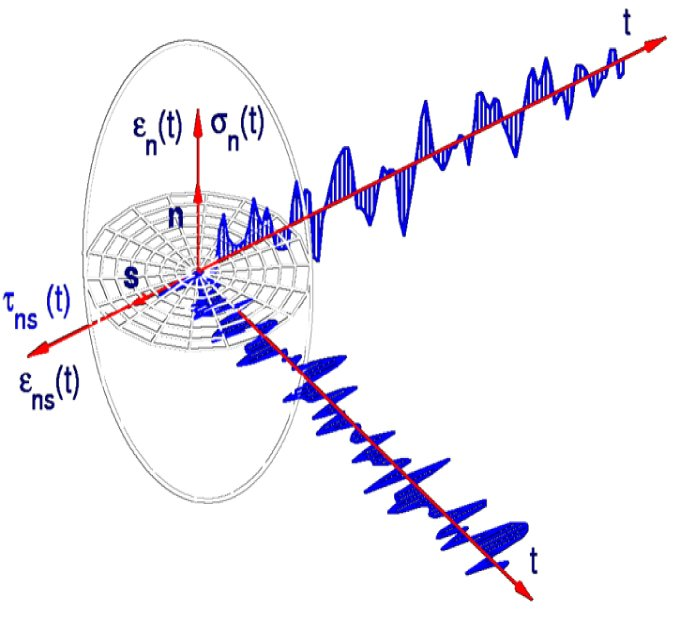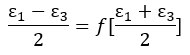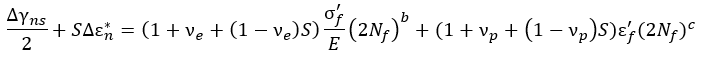# Multiaxial Fatigue

Multiaxial fatigue can be observed within many structures which are used in everyday life.  The effects of fatigue have to be evaluated using adapted models which consider some specific mechanisms.  Engineers are often surprised to see the large number of criteria.  There are so many different models available in this area.  These models are not only different because of the different types of equations they present, but also because of their critical criteria.Multiaxial fatigue tests can be performed allowing various stress states, such as biaxial traction states, torsion traction states, to be tested.  Tests simultaneously involving several loading modes are said to be in-phase or proportional if the main components of the stress or strain tensor simultaneously and respectively reach their maximum and minimum and their directions remain constant.  If it is not the case, they are said to be off-phase or non-proportional.

Many researchers have attempted to reduce multiaxial stress/strain state to uniaxial one, which is used in fatigue life calculation.  Such uniaxial parameter is often called ‘equivalent’ and it means that the same fatigue life is obtained under uniaxial (‘equivalent’) and multiaxial stress/strain state.  The reduction is based on the multiaxial fatigue failure criterion. Numerous multiaxial fatigue failure criteria have been proposed in recent decades.

Critical Plane Approach

The critical plane approach assumes that the fatigue failure of the material is due to some stress or/and strain components acting on the critical plane.  It is based upon the experimental observation that in metallic materials fatigue cracks initiate and grow on certain planes.  In this approach, the aspect of microdamage or even short crack propagation are not considered.  The critical plane approach concerns the crack initiation process that is usually related to fatigue failure at high cycle fatigue regime. However, it was successfully used also at low cycle fatigue regime.

Critical plane criteria reduce the multiaxial state of stress/strain to the equivalent-uniaxial state; this single damage parameter is used to calculate fatigue life or damage degree on a plane using the standard S-N curves.  Any phenomena regarding to the crack propagation are not considered.  Fatigue life to crack initiation is estimated.Findley Criterion

max(τa,n + kσn) = f

where τa,n is the shear amplitude on a plane with a unit normal n, i.e. τa,n = ∆τn/2 and σn is the normal stress on the same plane.  The two material coefficients k and f can be determined from fatigue loading tests.

The critical plane orientation coincides with the plane orientation where the maximum value of this linear combination occurs. It depends on the material coefficient k. Findley noticed that k value was small for ductile materials and the position of the critical plane for these materials approached to the direction of maximum shear stress.  A high k value is characteristic for brittle materials like cast iron, and the critical plane position is then compatible with the position of maximum principal stress direction σ1. Findley did not define a mathematical formula for the material coefficient f.  Some researchers assume that it can be determined from the shear-mode cracking.

McDiarmid Criterion

In the McDiarmid criterion, the critical plane is a plane with the maximum shear stress amplitude τmax.  To calculate the limit state, besides τmax, the effect of normal stress in the same plane σmax is considered. The mathematical criterion can take the following form

τmax / (τaf A,B) + σmax /(2σu) = 1

where τaf A and τaf B are torsion fatigue limits, for the case of an increase in cracking type A or B, and σu is a monotonic tensile strength. By transforming the formula above, we obtain a relationship defining the equivalent stress can be obtained:

σMD = τmax + kσmax ≤ τaf A,B

where

k = τaf A,B /(2σu)

Matake Criterion

According to Matake, the critical plane is one of two planes of maximum shear stress τns,a with a higher value of normal stress σn,max

τns,a + kσn,max ≤ τaf

Under this approach, there is only one coefficient in the criterion, which is determined using the following formula

k = 2 τaf / σaf − 1

Dang Van Criterion

Dang Van assumes that fatigue damage occurs with some grains which are not correctly oriented within the representative volume, which corresponds to some grains going through a plasticity phenomenon whereas the macroscopic stress does not reach elasticity limit and mainly remains in a elastic strain state.  He proposes a criterion which is based on a meso-macro switch, considering the stress distribution within the grains which get plasticized.  Dang Van’s criterion is based on a two-scale approach.  In metals the fatigue crack initiates at the grain level in the persistent slip bands (PSB) due to alternating shear stresses.  The tensile micro-scale hydrostatic stress will open the crack and accelerate its growth along the PSB.  The macroscale stresses which appear in the macroscale equilibrium equations are denoted as S and the micro-scale stresses appearing in the grains are denoted as σ.  The criterion for fatigue is postulated in the micro-scale as

τ (t) + aσh(t) = b

where τ (t) and σh(t) are the instantaneous shear and hydrostatic stresses on the slip band.  If τ (t) + aσh(t) < b, the structure will elastically shake-down to the applied loading.

The macroscale stress and microscale stress are related as

σ(t) = S(t) + dev ρ

where ρ is the stabilized residual stress tensor; dev = deviatoric part of.  During the elastic shakedown the microscale yield surface will expand and move according to isotropic and kinematic hardening laws and the stabilized residual stress tensor ρ designates the distance from the center of the yield surface from the origin. The state of shakedown is found if the stress path is completely inside the expanded and displaced yield surface.Dang Van Fatigue Failure Criterion in Micro-stress Meridian Plane

Kandil-Brown-Miller Criterion with Wang-Brown Modification

In 1973 Brown and Miller observed that fatigue cracks started and propagated on the maximum shearing plane while being helped by the stress normal to the plane.  In 1982 the Kandil-Brown-Miller criterion has been developed adopting a critical plane approach, justified by experimental observations of the nucleation and growth of cracks during loading: depending on the material, stress state, environment, and strain amplitude, fatigue life is usually dominated by crack growth along either shear planes or tensile planes.  Kandil, Brown and Miller proposed that both the cyclic shear and normal strain on the plane of maximum shear must be considered due to the fact that cyclic shear strains help to nucleate cracks, while normal strains contribute in their growth.

The criterion for multiaxial fatigue proposed by Brown and Miller is as follows:Or

γ13 = f[εn]

The assumption is that fatigue life is generally a non-linear function of the strain state.  The equation above represents the contour for a given fatigue life.

The critical plane is the plane with maximum shear strain γ13. This definition of critical plane is correct only in case of proportional loading assuming fixed principal strain directions. The criterion written in a general way above was reduced to a linear form by Kandil-Brown-Miller in 1982:Where S is a coefficient determined by experiments, called coefficient of normal strain influence.  Note that only in case of proportional loading, when shear strains and normal strains are in phase and their extremes occur at the same time instant, the application of strain ranges in the equation above is justified.  In order to take into account the influence of non-proportionality and variable-amplitude strains, Wang and Brown (1993) proposed to modify the criterion as follows:where

νe = Elastic Poisson’s ratio

νp = Plastic Poisson’s ratio

σ’f = Fatigue strength coefficient

b = Fatigue strength exponent

ε’f = Fatigue ductility coefficient

c = Fatigue ductility exponent

E = Modulus of elasticity

Nf = Fatigue life in number of cycles

Δε*n is called strain excursion; it is calculated in the plane of maximum shear strain range Δγns.  The range of shear strain is calculated in direction s in the plane with normal vector n.  If the beginning of the cycle is determined by point A and the end by point B, thenThe critical plane is one of the planes of maximum shear strain range Δγns with higher value of normal strain range Δε*n.  The critical plane is the plane with maximum fatigue damage.

Fatemi-Socie Criterion

Fatemi and Socie observing fatigue fractures came into conclusion similar to those by Brown and Miller, that is, the normal strain εn in the plane of maximum shear strain accelerates the fatigue damage process through crack opening.  Crack opening decreases the friction force between slip planes blocking crack growth.  Hence, normal stress σ in the plane of maximum shear strain amplitude γ was introduced in the fatigue model.This model was born as a modification of the Brown-Miller’s critical plane model, considering mainly the maximum shear strain amplitude and the maximum normal stress on the maximum shear strain amplitude plane. Fatemi and Socie noticed that Brown and Miller criterion does not include additional material hardening occurring under non-proportional loading.  In order to take into material hardening effect, they modified the Brown-Miller criterion by replacing the value of normal strain εn  in the critical plane with the maximum normal stress value σn,max.  The critical plane is the plane of maximum shear strain amplitude γns,a.  For a given number of cycles to failure Nf, the proposed criterion is given by

γns,a(1 + k σn,maxy) = constant

where k is the normal stress sensitivity coefficient.  This parameter describes the influence of normal stress on fatigue life.  The tested k values for 304 and 316 stainless steels are as shown in the following chart:The following chart is a k-N relationship for an HSLA steel with E = 210 GPa:If it is difficult to get a k value, it would be safely taken as 1.0.

In terms of maximum shear strain amplitude γns,a, the Fatemi-Socie criterion is given byor in terms of strain range Δγmax the equation can be rewritten aswhere

γns,a   = Maximum shear strain amplitude

Δγmax = Maximum shear strain range

k = Normal stress sensitivity coefficient

σn,max = Maximum normal stress value on maximum shear strain amplitude

σy = Yield strength

νe = Elastic Poisson’s ratio

νp = Plastic Poisson’s ratio

σ’f = Fatigue strength coefficient

b = Fatigue strength exponent

ε’f = Fatigue ductility coefficient

c = Fatigue ductility exponent

E = Modulus of elasticity

Nf = Fatigue life in number of cycles